Home > CC4 > Chapter 11 > Lesson 11.2.5 > Problem11-107

11-107.
1. Write an equation in standard form for each of the following sequences. Homework Help ✎

2.  a.,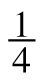,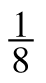, ... b. −7.5, –9.5, −11.5, …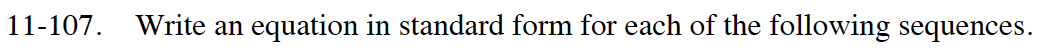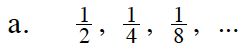Look at the first number in the sequence and determine how much each following term increases or decreases.

$\text{In this case, }\frac{1}{2} \text{ is the first term.}$

$\text{The terms decrease by a factor of }\frac{1}{2}.$

$\textit{t}(\textit{n})=\frac{1}{2}\left(\frac{1}{2}\right)^{\textit{n}-1}$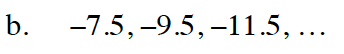Note that each term decreases by 2.